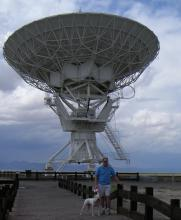# UBV Transformations for SRO50

8 posts / 0 new
HQAUBV Transformations for SRO50

Here are the transformations from this season:

Tv [B-V] = -0.011 +/- 0.005

Tbv = 1.005 +/- 0.015

Tub = 1.179 +/- 0.035

(U-B) is nonlinear, but these values should be ok for AZ Cas.

Arne

WWAAThank you so much

Thank you so much Arne!

Can these please be uploaded to VPHOT for SRO50?  Otherwise I have to re-enter them for every transition I do.

Thanks!

Wayne

WWAAWhen I do the UB

When I do the UB transformation, VPHOT is aksing for a Tu value. What do I use?

WWAAAnd can these new values please be uploaded to VPHOT for SRO50?

My work on the AZ Cas project is still on hold.

Thanks!

Wayne

HQASRO50 transformation coefficients

Since VPHOT transforms magnitudes only and not colors, it looks for Tu, Tb, Tv instead of Tv, Tbv, Tub.  So I needed to calculate two additional coefficients: Tu  (U as a function of U-B) and Tb (B as a function of B-V).  These are:

Tu [U-B] = 0.153

Tb [B-V] = 0.028

plus the original

Tv [B-V] = -0.011

That should give enough information for calculating U,B,V from Sonoita data.

Arne

MGQ
SRO50 UBV transformation coefficients

Hi Arne,

On November 7, 2012 - 10:31am You posted

*************

Here are the transformations from this season:

Tv [B-V] = -0.011 +/- 0.005

Tbv = 1.005 +/- 0.015

Tub = 1.179 +/- 0.035

Tb should then = 1.005 + (-0.011) + 0.994

and

Tu should then = 1.179 + 0.994 = 2.173 which is not good at all.

Posted: December 22, 2012 - 11:23am

Since VPHOT transforms magnitudes only and not colors, it looks for Tu, Tb, Tv instead of Tv, Tbv, Tub.  So I needed to calculate two additional coefficients: Tu  (U as a function of U-B) and Tb (B as a function of B-V).  These are:

Tu [U-V] = 0.153

Tb [B-V] = 0.028

plus the original

Tv [B-V] = -0.011

*************

This means Tbv = 0.028 - (-0.022) = 0.039
and Tub = 0.153 - 0.039 = 0.114

As I recall from my photon counting days Tv (epsilon) should be close to zero and Tbv (mu) close to 1.0 and Tub (osi) close to 1.0.

There seems to be some conflicting information or maybe old age has dimmed my reasonng. Can you please set things straight as something does not seem correct. I hope we can get this straight as we have a lot of images for AZ Cas ready for data extraction and reduction.

Jeff HPO (187283)

HQATbv, Tub

Hi Jeff,

You are making a basic mistake, equating Tbv to Tb - Tv.  The full equations are given in Henden and Kaitchuck, and these two color coefficients were given in my first posting (and are close to 1.0, as you expect).  The AID requires that you submit data in magnitude form - that is, U, B, V - rather than the magnitude/color form found in professional literature - V, (B-V), (U-B).  Because of this, you need three magnitude coefficients for U,B and V in order to submit those magnitudes as transformed values.

When I do my own photometry, I typically obtain V, (B-V), (U-B) as transformed values, and then submit the magnitudes by proper addition and subtraction of the color indices from V.  So for my photometry, I use Tv plus Tbv, Tub.  However, VPHOT is not set up that way (yet).

Arne

MGQ
Tbv, Tub

Hi Arne,

Thanks. I figured it might be something like that. I always use the epsilon, mu and psi for my UBV reduction. I have never used the Tb and Tu coeffieicents.

Jeff